# LINEAR PROGRAMMING

Linear Programming is mathematical modelling of the real world problems that can be solved by quantative methods where decision variables are unknowns to be obtained, objective/goal function is formulation of decision variables, optimal solution is the result of the objective function which is the optimal value and constraints are the physical limitations of the system. The main assumptions of a linear programming problems are;

• There is a Single objective; minimization or maximization
• All variables are continues
• Objective and constraints are linear
• All decision variables are nonnegative (as negative is not possible in real world).

There are several methods to solve the linear programming problems; Simplex, Criss-cross, Ellipsoid, Protective, etc. Simplex is a popular algorithm. It has iterative approach by improving the value of the objective function at each step.

Simplex (Standard) (see Example-1)

Main steps of Simplex solution;

• Establishing a mathematical model of the real world problem (representing as formulas)
• Checking negative RHS (Right Hand Sides). If any, correct it by multiplying all coefficient by -1 and changing the inequality type. For example; 1x1 - 2x2 <= -5  becomes -1x1 + x2 >= 5 after correction.
• Transforming the inequalities to equlaties for constraints (Standard Form - representing as formulas). In most of the cases, constraints are in inequality form. Therefore, they need to be trasformed to the equality form.
• Decision variables are named as Non Basic Variables (default legend is “x”) while others are named as Basic variables. Basic variables include slack variables, artificial variables and extra variables. The basic variables are needed to transform a problem to standard form;

• Slack variables are added for <= constraints with default legend “s”
• Artificial variables are added for = and >= constraints with default legend “a”
• Extra variables are added for >= constraints with default legend “e”

• Creating initial matrix from Standard Form.
• Selecting the variable to enter (incoming variable)
• Selecting the variable to leave (outgoing variable)
• Pivoting
• Checking feasibility and degeneration
• Repeat the steps or terminate

There are four possibilities at the end;

• Single optimal solution, Best Feasable Solution (BFS), OPTIMAL
• Multiple solutions; ALTERNATIVES
• No feasable solution; INFEASABLE
• infinite number of solution; UNBOUNDED

Two Phase Simplex

If all constraints are in <= form, the problem can be solved in one phase as outlined above. If even one contraint is in “>=” or “=” form, two phase Simplex rules are followed. In the first phase, an auxiliary problem created from the problem is solved first to find out if it is feasable or not. If it is feasable, orijinal objective function is taken into the calculations in the second phase.

Revised Simplex (see Example-2)

The Revised Simplex is different implementation of the Simplex. It provides computational effectiveness and less memory usage for the large problems. The Revised Simplex follows the Simplex Solution steps but initial table is an Unity Matrix at the beginning.

One phase and two phase Revised Simplex methodes are same as Simplex.

Dual Problem

A linear programming problem is a Primal problem. It can be converted to Dual of the problem to obtain the upper bound of the Primal’s optimal.

Main steps of converting to Dual:

• Taking transpose of Constraints Coeffs matrix (excluding RHS column)
• Converting “>=” to “<=” or vise versa
• Interchanging Objective function Coeffs and RHS values

After that, the Dual Problem is solved by Simplex or Revised Simplex (both one phase or two phase)

Implementation in SimpleLPsolver

Simplex Solver (see Example-1)

The simplex method for maximization problems is used. Therefore, minimization problems are converted to maximization problems. Following steps are followed;

• Check the goal function for zero coefficients for all variables.
• Check and fix the constraints for zero coefficients for all variables.
• Check and fix redundant constraints.
• Check and fix negative RHS values.
• Continue with Single Phase procedure if all constraints are <=, otherwise switch to Two Phase procedure.
• Single Phase Simplex procedure;
• Add slack variables (s) to bring all constraints into equality ( =) form.
• Create initial simplex table as shown below;
 x1 x2 ... e1 e2 ... s1 s2 ... Z 0 Const.1 RHS 1 Const.2 RHS 2 Const.3 RHS 3 ... ...
• Solve the problem in accordance with maximization problem criterias.
• Two Phase Simplex procedure;
• Add slack variables (s), artificial variables (a) and excess variables (e)  to bring all constraints into equality ( =) form.
• Create initial two phase simplex table as shown below;
 W x1 x2 ... e1 e2 ... s1 s2 ... a1 a2 ... w 0 Z 1 Const.1 RHS 1 Const.2 RHS 2 Const.3 RHS 3 ... ...
• Calculate the initial values for w row for Basic Feasible Solution (BFS).  Z row is not needed for this stage but it is useful since original objective function will be in the appropriate form for the second phase.
• Solve the problem in accordance with maximization problem criterias as Phase 1.
• If the BFS is found at the end of Phase 1, eliminate w row and artificial variables from the table.
• Continue to solve the problem in accordance with maximization problem criterias as Phase 2.

Revised Simplex Solver (see Example-2)

The revised simplex method for minimization problems is used. Therefore, maximization problems are converted to minimization problems. Following steps are followed;

• Check the goal function for zero coefficients for all variables.
• Check and fix the constraints for zero coefficients for all variables.
• Check and fix redundant constraints.
• Check and fix negative RHS values.
• Continue with Single Phase procedure if all constraints are <=, otherwise switch to Two Phase procedure.
• Single Phase Simplex procedure;
• Add slack variables (s) to bring all constraints into equality ( =) form.
• Create initial revised simplex table as shown below;
 x1 x2 x3 ... Z 0 Const.1 RHS 1 Const.2 RHS 2 Const.3 RHS 3 ... ...
• Create Basis Inverse (B-1 ) matrix with artificial variables as shown below (initially unity matrix);

 z s1 s2 s3 ... z 1 s1 1 s2 1 s3 1 ... 1
• Solve the problem in accordance with minimization problem criterias.
• Two Phase Revised Simplex procedure;
• Add slack variables (s), artificial variables (a) and excess variables (e)  to bring all constraints into equality ( =) form.
• Create initial two phase revised simplex table as shown below;
 w x1 x2 x3 ... e1 e2 ... w 0 z 1 Const.1 RHS 1 Const.2 RHS 2 Const.3 RHS 3 ... ...
• Create Basis Inverse (B-1 ) matrix with artificial variables as shown below (initially unity matrix);
 w z s1 s2 s3 ... a1 a2 a3 ... w 1 z 1 s1 1 s2 1 s3 1 ... 1 a1 1 a2 1 a3 1 ... 1
• Calculate the initial values for w row for Basic Feasible Solution (BFS).  Z row is not needed for this stage but it is useful since original objective function will be in the appropriate form for the second phase.
• Solve the problem in accordance with maximization problem criterias as Phase 1.
• If the BFS is found at the end of Phase 1, eliminate w row-column and artificial variables from the table.
• Continue to solve the problem in accordance with maximization problem criterias as Phase 2.

Dual Simplex Solver

Finds the Dual problem by taking transpose of Constraints Coeffs matrix (excluding RHS column), converting “>=” to “<=” or vise versa and interchanging Objective function Coeffs and RHS values.

After that, the Dual Problem is solved by Simplex (both one phase or two phase) method as described above.

Dual Revised Simplex Solver

Finds the Dual problem by taking transpose of Constraints Coeffs matrix (excluding RHS column), converting “>=” to “<=” or vise versa and interchanging Objective function Coeffs and RHS values.

After that, the Dual Problem is solved by Revised Simplex (both one phase or two phase) method as described above.

Guestbook
 *Name E-mail *Comment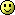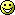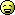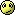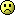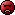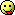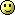Fields marked with an asterisk * are required.
Pages: 1 2 3 (3)

Name: oratuit
E-mail: squeelp@abdiell.xyz
Sent: 07/24/2021 05:23:05 am

Name: oratuit
E-mail: squeelp@abdiell.xyz
Sent: 07/10/2021 01:42:31 am

<a href=https/vsaccutanev.com/>accutane online fast deliverey</a>
Name: oratuit
E-mail: squeelp@abdiell.xyz
Sent: 06/27/2021 10:27:57 am

<a href=https/priligyset.com>viagra dapoxetine online purchase
Name: oratuit
E-mail: squeelp@abdiell.xyz
Sent: 06/15/2021 04:41:49 pm

<a href=https/vsantabusev.com>buy antabuse
Name: oratuit
E-mail: squeelp@abdiell.xyz
Sent: 05/30/2021 08:43:09 am

Name: unawuxsocotut

Sent: 05/21/2021 11:07:20 am

http://slkjfdf.net/ - Acoateat <a href="http://slkjfdf.net/">Efakihi</a> unu.uhkq.tanels.com.zhm.wm http://slkjfdf.net/
Name: acowumejicume

Sent: 05/21/2021 10:57:39 am

http://slkjfdf.net/ - Atixag <a href="http://slkjfdf.net/">Azoxaa</a> eea.ltar.tanels.com.qsr.ps http://slkjfdf.net/
Name: ufihoxmqwume
E-mail: efepol@obowi.jonjamail.com
Sent: 05/15/2021 06:15:14 am

http://slkjfdf.net/ - Uretagete <a href="http://slkjfdf.net/">Ikaxugame</a> oxk.rjsz.tanels.com.crg.tg http://slkjfdf.net/
Name: ihamehia
E-mail: aqewod@obowi.jonjamail.com
Sent: 05/15/2021 06:07:22 am

http://slkjfdf.net/ - Ufatpi <a href="http://slkjfdf.net/">Akapit</a> npz.fhxb.tanels.com.rty.jx http://slkjfdf.net/
Name: zukavuraxuno

Sent: 05/15/2021 05:59:59 am

http://slkjfdf.net/ - Igejupice <a href="http://slkjfdf.net/">Umalus</a> slm.hdga.tanels.com.cdt.wu http://slkjfdf.net/